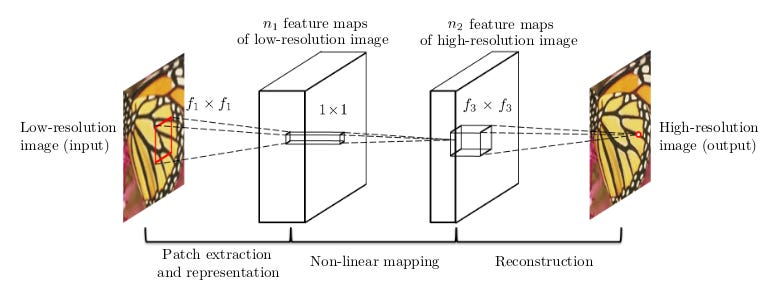# Superresolution explained — Ep2

Source: Deep Learning on Medium

This article is a part of a series of articles. Please check this article for the problem definition and general rubrics.

In this article we will discuss the first method, SRCNN. It was one of the first to formally use deep learning to solve the problem of SR.

### Approach I: SRCNN

SRCNN , also known as Super Resolution Convolutional Neural Network, was among the early few papers to use Deep Learning, especially CNNs to solve the problem of SR. It was developed by a team from MMLAB in CUHK. The main paper can be found here.

Note: The notation in the paper interchanges X and Y w.r.t our definition. Using X for input is more intuitive to understand. Hence the change.

#### Network:

The method used in SRCNN has 3 sub networks/layers. It does not involve any up-scaling by neural networks but instead uses classical interpolation techniques to upscale and improves the resultant quality by a CNN.

Prior to passing through Convolutional Networks, the small image (x) is enlarged to the required factor by Bicubic/Spline Interpolation. This image (X) is the input (referred still as “Low Resolution”) and the “Ground Truth” SR image Y (also, called Y_true) is the output such that a mapping F(X) = Y is possible.

The figure below gives an overview of the network. It will be further explained later. Low resolution now refers to the interpolated image.An overview of the Neural Network pipeline. Input represents interpolated image and output represents the high resolution image. Each layer of the CNN serves a very specific purpose 

Step I — Patch Extraction

From the large image (interpolated), the first layer acts as a patch extractor such that each (c ×f1× f1) dimensional region of the image is mapped as one 1 x 1 point in the n1 dimensional feature space. This is done using a convolutional layer (weights W1, bias B1); with n1 filters each and having a kernel size f1 is passed on the image. The mapping is activated with a Rectified Linear Unit (ReLU, max(0,x)). We refer the entire mapping as F1(X). Mathematically the convolution is shown as —

This gives us n1 different feature maps for the low resolution image, that can now be mapped further for a finer quality image.

Step II — Non Linear Mapping

The first layer extracts an n1-dimensional feature for each patch. In this layer we map these features F1(X) to another feature map F2(F1(X)) using a convolutional layer with weights W2, bias B2 and kernel size f2. This is also activated using a ReLU activation. Mathematically,

Here W2 is of a size n1×1×1×n2, and B2 is n2-dimensional. Each of the output n2-dimensional vectors is is a representation of the high resolution image output, which is ready for reconstruction.

We can add more such layers to improve on the accuracy but the computation cost s accuracy trade-off may not be favorable.

Step III — Reconstruction

In this step we want to generate the final image. The reconstruction layer convolves over the feature map of the previous layer to change the depth to c, the number of channels. It uses weights W3 and bias B3 while the filter size is set to f3. Thus —

Here W3 has size (n2×f3×f3×c), and B3 is a c dimensional vector. This brings the image to c number of channels.

Note: In all convolution operations in this algorithm, the image is padded in the ‘same’ configuration, i.e. the zeros are always padded along the image such that the convolution output dimensions equal the input.

All such patches are then added to make the final image. Hence, in regions where one pixel is a part of more than one patches, the average of the value of that pixel in all such patches is taken as the final value.

Training:

The above 3 layer network comprises the SRCNN network with each layer driven by a different intuition. For training this network, we use the parameters as n1=64, n2=32, f1=9, f2=1 and f3=5.

This is inspired from the previous sparse coding approaches. It is however an experimental value and can be changed as per dataset or convergence graphs of the loss function.

Loss Function — Mean Squared Error

For each patch Xi, we evaluate F(Xi) = Yi for the given network. This gives us Yi’s of different patches in the images. We want to update the weights such that minimize the loss function or the optimum goal.

In this network configuration, Mean Squared Error (MSE) as defined above is used as the optimum goal. That is, the mean squared error in the generated and expected image should be the least.

Optimizer — Gradient Descent

We want to update weights to reduce the loss. This is done by moving in the direction of maximum slope in the weight dimension. This descent method is called gradient descent.

When we update the weights using Gradient Descent after each sample, the optimization method is called stochastic gradient descent. If we update it after every batch of data points, it is called Batch Gradient Descent.

Dataset — T91, Set5, Set14

The T91 dataset contains 91 images. Set5 and Set14 contain 5 and 14 images respectively. We use T91 for training while the other two for testing.

We extract small patches using the network itself to run on it. We experiment for values of k = 2,3 and 4.

However, we can use data from the BSDS dataset for training and even augment the existing data by flipping or rotating the images/patches.

Code:

Multiple implementations of the algorithm can be found on github. The SRCNN page itself provides implementations in Matlab and Caffe.

Explanations of code and helpers can also be found at https://github.com/manasbedmutha98/SRCNN

References:

 Chao Dong, Chen Change Loy, Kaiming He, Xiaoou Tang, “Image Super-Resolution Using Deep Convolutional Networks”, http://arxiv.org/abs/1501.00092

Stay tuned for more content! Let me know your thoughts on the article in the comments or here.